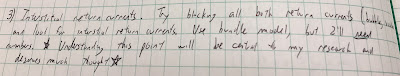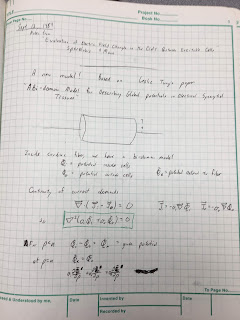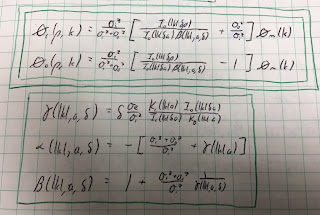## Friday, October 5, 2018

### A Bidomain Model for the Extracellular Potential and Magnetic Field of Cardiac TissueMy research notebooks from graduate school.
In graduate school, I worked with John Wikswo measuring the magnetic field of a nerve axon. We isolated a crayfish axon and threaded it through a wire-wound ferrite-core toroid immersed in saline. As the action currents propagated by, they produced a changing magnetic field that induced a signal in the toroid by Faraday induction. Ampere’s law tells us that the signal is proportional to the net current through the toroid, which is the sum of the intracellular current and the fraction of the current in the saline that passes through the toroid, called the return current.

For my PhD dissertation, Wikswo had me make similar measurements on strands of cardiac tissue, such as a papillary muscle. The instrumentation was the same as for the nerve, but the interpretation was different. Now the signal had three sources: the intracellular current, the return current in the saline, and extracellular current passing through the interstitial space within the muscle called the “interstitial return current.” Initially neither Wikswo nor I knew how to calculate the interstitial return current, so we were not sure how to interpret our results. As I planned these experiments, I recorded my thoughts in my research notebook. The July 26, 1984 entry stressed that “Understanding this point [the role of interstitial return currents] will be central to my research and deserves much thought.”Excerpt from the July 26, 1984 entry in my Notebook 8, page 64.
When working on nerves, I had studied articles by Robert Plonsey and John Clark, in which they calculated the extracellular potential in the saline from the measured voltage across the axon’s membrane: the transmembrane potential. I was impressed by this calculation, which involved Fourier transforms and Bessel functions (see Chapter 7, Problem 30, in Intermediate Physics for Medicine and Biology). I had used their result to calculate the magnetic field around an axon (see Chapter 8, Problem 16 in IPMB), so I set out to extend their analysis again to include interstitial return currents.Page 1 of Notebook 9, from Sept 13, 1984.
The key was to use the then-new bidomain model, which accounts for currents in both the intracellular and interstitial spaces. The crucial advance came in September 1984 after I read a copy of Les Tung’s PhD dissertation that Wikswo had loaned me. After four days of intense work, I had solved the problem. My results looked a lot like those of Clark and Plonsey, except for a few strategically placed additional factors and extra terms.Excerpt from Notebook 9, page 13, the Sept 16, 1984 entry.First page of Roth and Wikswo (1986).
Wikswo and I published these results as a brief communication in the IEEE Transactions on Biomedical Engineering.
Roth, B. J. and J. P. Wikswo, Jr., 1986, A bidomain model for the extracellular potential and magnetic field of cardiac tissue. IEEE Trans. Biomed. Eng., 33:467-469.

Abstract—In this brief communication, a bidomain volume conductor model is developed to represent cardiac muscle strands, enabling the magnetic field and extracellular potential to be calculated from the cardiac transmembrane potential. The model accounts for all action currents, including the interstitial current between the cardiac cells, and thereby allows quantitative interpretation of magnetic measurements of cardiac muscle.
Rather than explain the calculation in all its gory detail, I will ask you to solve it in a new homework problem.
Section 7.9
Problem 31½. A cylindrical strand of cardiac tissue, of radius a, is immersed in a saline bath. Cardiac tissue is a bidomain with anisotropic intracellular and interstitial conductivities σir, σiz, σor, and σoz, and saline is a monodomain volume conductor with isotropic conductivity σe. The intracellular and interstitial potentials are Vi and Vo, and the saline potential is Ve.
a) Write the bidomain equations, Eqs. 7.44a and 7.44b, for Vi and Vo in cylindrical coordinates (r,z). Add the two equations.
b) Assume Vi = σiz/(σiz+σoz) [A I0(kλr) + (σoz/σiz) Vm] sin(kz) and Vo = σiz/(σiz+σoz) [A I0(kλr) - Vm] sin(kz) where λ2 = (σiz+σoz)/(σir+σor). Verify that Vi - Vo equals the transmembrane potential Vm sin(kz). Show that Vi and Vo obey the equation derived in part a). I0(x) is the modified Bessel function of the first kind of order zero, which obeys the modified Bessel equation d2y/dx2 + (1/x) dy/dx = y. Assume Vm is independent of r.
c) Write Laplace’s equation (Eq. 7.38) in cylindrical coordinates. Assume Ve = B K0(kr) sin(kz). Show that Ve satisfies Laplace’s equation. K0(x) is the modified Bessel function of the second kind of order zero, which obeys the modified Bessel equation.
d) At r = a, the boundary conditions are Vo = Ve and σirVi/∂r + σorVo/∂r = σeVe/∂r. Determine A and B. You may need the Bessel function identities dI0/dx = I1 and dK0/dx = - K1, where I1(x) and K1(x) are modified Bessel functions of order one.
In the problem above, I assumed the potentials vary sinusoidally with z, but any waveform can be expressed as a superposition of sines and cosines (Fourier analysis) so this is not as restrictive as it seems.

In part b), I assumed the transmembrane potential was not a function of r. There is little data supporting this assumption, but I was stuck without it. Another assumption I could have used was equal anisotropy ratios, but I didn’t want to do that (and initially I didn’t realize it provided an alternative path to the solution).

The calculation of the magnetic field is not included in the new problem; it requires differentiating Vi, Vo, and Ve to find the current density, and then integrating the current to find the magnetic field via Ampere’s law. You can find the details in our IEEE TBME communication.

Some of you might be thinking “this is a nice homework problem, but how did you get those weird expressions for the intracellular and interstitial potentials used in part b)”? Our article gives some insight, and my notebook provides more. Basically I started with Clark and Plonsey’s result, used ideas from Tung’s dissertation, and then played with the math (trial and error) until I had a solution that obeyed the bidomain equations. Some might call that a strange way to do science, but it worked for me.

I was very proud of this calculation (and still am). It played a role in the development of the bidomain model, which is now considered the state-of-the-art model for simulating the heart during defibrillation.

Wikswo and I carried out experiments on guinea pig papillary muscles to test the calculation, but the cardiac data was not as clean and definitive as our nerve data. We published it as a chapter in Cell Interactions and Gap Junctions (1989). The cardiac work made up most of my PhD dissertation. Vanderbilt let me include our three-page IEEE TBME communication as an appendix. It’s the most important three pages in the dissertation.

Coda: While browsing through my old research notebooks, I found this gem passed from Prof. Wikswo to his earnest but naive graduate student, who dutifully wrote it down in his research notebook for posterity.Excerpt from Notebook 10, Page 62.